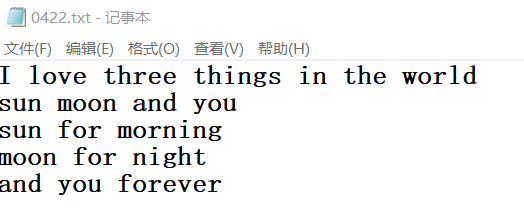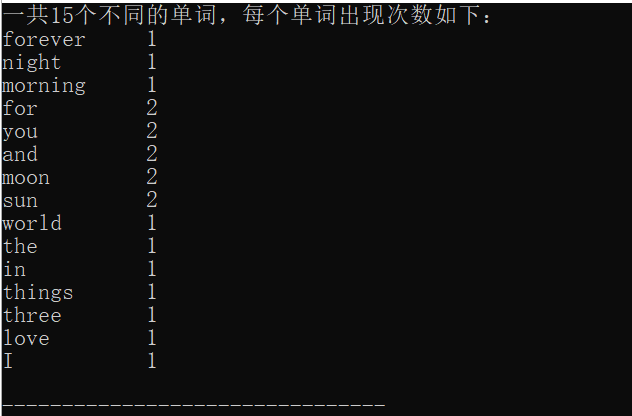• 1.从输入流接收或给定的字符数组中，统计单词的出现次数。 2.我的代码 如下：请高手帮忙指教是哪里出了问题？感谢！ void count_words() { char text = { "on","the","desk","on","frank","the","hello" ...
• c语言 英文单词频率统计 哈希存储 从一段英文文章中找到频率最大的几个单词，同时统计不同单词个数以及总单词个数，利用哈希进行存储。 #include<stdio.h> #include<ctype.h> #include<string.h> ...
c语言 英文单词频率统计 哈希存储
从一段英文文章中找到频率最大的几个单词，同时统计不同单词个数以及总单词个数，利用哈希进行存储。
#include<stdio.h>
#include<ctype.h>
#include<string.h>
#include<stdlib.h>
#include <stdio.h>
#include <stdlib.h>

#define HASH_TABLE_SIZE 49999

typedef struct word{
char wrd;
int num;
}word;
static int diff_words=0;
static int total_words=0;

word *hashtable[HASH_TABLE_SIZE];

int hash(int number){
return number%HASH_TABLE_SIZE;
}
void insert(int count,char *w)
{
int pos=hash(count);
word *newWord;
total_words++;
while (hashtable[pos])
{
if(!strcmp(hashtable[pos]->wrd,w))
{
hashtable[pos]->num++;
return;
}
else
{
pos++;
}
}
newWord=(word*)malloc(sizeof(word));
newWord->num=1;
strcpy(newWord->wrd,w);
hashtable[pos]=newWord;
diff_words++;
}

int main()
{
FILE *fp;
char wrd_temp,ch;
word *head,*curr;
int i,arr,count=0,j;

word *temp=(word*)malloc(sizeof(word));
memset(hashtable,0,HASH_TABLE_SIZE);
fp=fopen("TheGun.txt","r");   //只读方式打开
i=0;
while (1)
{
ch=fgetc(fp);
if(isalpha(ch)||ch=='\'')
{
if(islower(ch))
{
wrd_temp[i++]=ch-32;  //小写字母转大写
count=count+ch-32;  //count用于计算单词的哈希值
}
else
{
wrd_temp[i++]=ch;
count=count+ch;
}
}
else
{
wrd_temp[i++]='\0';
if(strlen(wrd_temp)>=1)
{
insert(count,wrd_temp);
}
memset(wrd_temp,0,80);
i=0;
count=0;
}
if(feof(fp))
break;
}
fclose(fp);
printf("Number of total_words = %d\n",total_words);
printf("Number of different words = %d\n",diff_words);
printf("The 100 most common words:\n");
printf("WORD            NUMBER OF OCCURRENCES\n");
for (i = 0; i < 10; i++)
{
count=0;
for (j = 0; j < HASH_TABLE_SIZE; j++)
{
if(hashtable[j]!=NULL && hashtable[j]->num>count)
{
count=hashtable[j]->num;
arr[i]=j;
}
}
printf("%s %d\n",hashtable[arr[i]]->wrd,hashtable[arr[i]]->num);
hashtable[arr[i]]->num=0;
}
return 0;
}


效果图展开全文哈希
• 从一文本中读取单词统计其出现的频率，并按频率大小输出到另一文本中。这里的单词是分二种，一种是ASCII码大于0x20小于0X80字符，第 二种是小于0x20以及大于0x80的字符。这里我用的是哈希表，这样能很快查找读取的...
从一文本中读取单词，统计其出现的频率，并按频率大小输出到另一文本中。这里的单词是分二种，一种是ASCII码大于0x20小于0X80字符，第 二种是小于0x20以及大于0x80的字符。这里我用的是哈希表，这样能很快查找读取的单词是否已经存在。排序我用的是二个循环，按理来说可以改进。
下面是我的算法。有些地方还需要改进。

#include <stdio.h>
#include <stdlib.h>
#include <string.h>

#define  FOOL     int
#define  TURE      1
#define  FALSE     0
#define  MAXWORD   256
#define  hashnum  1024

/*采用哈希表结点结构*/
typedef struct Hash{
int count;
char str;     /*不一定为1，而是动态分配*/
}Hash_table;

Hash_table hash_table[hashnum];

char buffer;     /*缓冲区大小，用来暂时存储单词*/
int tail_prob;              /*缓冲区结束位指针 */
int Hcount = 0;                   /*用来计算有多少单词*/

/******************************************************************/
/**                      初始化操作                              **/
/**                                                              **/
void initial_buffer ()
{
for (tail_prob = 0; tail_prob < MAXWORD ;tail_prob++)
{
buffer[tail_prob++] = 0;
}
tail_prob = 0;
}

void initial_hashtable ()
{
unsigned int i;
i = 0;
while (i < hashnum)
{
hash_table[i].count = 0;
i++;
}
}
/******************************************************************/
/**    关于缓冲区的操作                                          **/
/**                                                              **/

/*把一个字符拷贝到缓存区中*/
void copytobuffer (char character)
{
if (tail_prob >= MAXWORD)
{
printf("Usag:this word is too long!");
exit (-1);
}
buffer[tail_prob++] = character;
}

/*清除缓冲区*/
void clear_buffer ()
{
while (tail_prob != 0)
{
buffer[--tail_prob] = 0;
}
}

/**************************************************************/
/** 找哈希表的关键字,这里使用的是把字符串的值相加再求与哈希表**/
/**长度的余                                                  **/
unsigned int get_key (char *str)
{
unsigned result = 0;
do
{
result = result + (*str);
str++;
}while (*str != NULL);
result = result % hashnum;
return result;
}

/***************************************************************/
/**看哈希表中是否有对应的单词，有返回真                       **/
/**                                                           **/
int find_match ()
{
int i;
char *str;
char *temp;
int len1;          /*缓冲区中单词的长度*/
int len2;          /*哈希表中单词的长度*/
int index;

str = buffer;
index = get_key (buffer);
temp = hash_table[index].str;
len1 = strlen (buffer);
len2 = strlen (temp);

while (hash_table[index].count)
{
if (len1 == len2)     /*些比较len1和len2是否相等，如果不等再比较*/
{
for (i = 0;i<len1 && *str == *temp;i++)
{
str++;
temp++;
}
if (i != len2)
{
index = (index + 1) % hashnum;
temp = hash_table[index].str;
str = buffer;
}
else   //能找到
{
hash_table[index].count++;
return TRUE;
}
}
else
{
index = (index + 1) % hashnum;
temp = hash_table[index].str;
str = buffer;
}
}
}

/***************************************************************/
/** 根据缓冲区里面的字符生成哈希表                            **/
/**                                                           **/
void addtoHash()
{
char str_temp;
char *str;
char *temp;
int len;
unsigned int index;
int i;

i=0;
str = str_temp;
strcpy (str,buffer);
index = get_key (str);
len = strlen(str);
temp = hash_table[index].str;

while (hash_table[index].count)  /*找到没有被储存的结点*/
{
index = (index + 1) % hashnum;
}
hash_table[index].count++;
while (i < len)  /*复制字符串*/
{
*temp++ = *str++;
i++;
}
}

/***************************************************************/
/**                 排序后输出到out文件中                     **/
/**                                                           **/
void Hash_sort(FILE *out)
{
int index;
unsigned int symbol; /*最小值*/
int num;
int len;
int i = 0;
index = 0;

/*排序输出到out文件中,共有Hcount个单词*/
while (i < Hcount)
{
/*找到第一个遇见的频率非零的结点*/
while (hash_table[index].count == 0)
{
index ++;
}
symbol = hash_table[index].count;  /*充当最小值*/
num = index;
index++;
while (index < hashnum)
{
if (hash_table[index].count < symbol && hash_table[index].count)
{
symbol = hash_table[index].count;
num = index;
}
index ++;
}
/*找到了最小值*/
len = strlen (hash_table[num].str);
printf("%d ",hash_table[num].count);
printf("%s/n",hash_table[num].str);
fprintf(out,"%s,%d/n",hash_table[num].str,hash_table[num].count);

hash_table[num].count = 0;
i++;
index = 0;
}
}

/*****************************************************************
/*找文件in中单词出现的频率，存储其单词以及其频率，调用find_match*/
/*输入的字符小于等于Ox20或者大于0x80时说明不是数字或者字母。但是*/
/*也能组成一个单词                                              */
void find_frquence(FILE *in,FILE *out)
{
char character;
FOOL flag;
flag = 1; /*开关语句*/

initial_buffer();
initial_hashtable ();

/*当没有到文件结束时*/
character = fgetc (in);
do
{
if (character >0x20 && character < 0x80)
{
if (flag)
{
copytobuffer (character);
}
else /*新单词，且是字母和数字的组合*/
{
buffer[tail_prob] = '/0';/*表示结束*/
if(find_match ())  /*如果能够找到，相对应的频率加1*/
{
//     Tprob->count++;
}
else             /*不能找到，则生成一个新的结点加入孩子兄弟链表中*/
{
addtoHash ();
Hcount++;
}
clear_buffer ();
copytobuffer (character);
flag = !flag;
}
}
else
{
if (character <= 0x20 || character >= 0x80)
{
if (flag)
{
buffer[tail_prob] = '/0';
if (find_match ())   /*如果能够找到，则对应的频率加1,find_match实现频率加1*/
{
//      Tprob->count++;
}
else                 /*不能找到，则到哈希表中*/
{
addtoHash ();
Hcount++;
}
clear_buffer ();   /*清除缓冲区*/
copytobuffer (character);
flag = !flag;
}
else
{
copytobuffer (character);
}
}
}// if - else 结束
}while ((character = fgetc (in)) != EOF);

/*处理最后缓冲区所存储的单词*/
if(find_match())
{
//  Tprob->count++;
}
else
{
addtoHash();
Hcount++;
}

/*排序并按从小到大输出到out文件中*/
Hash_sort(out);
}

/**************************************************************/

void main(int argc,char *argv[])
{
FILE *in;
FILE *out;
char temp_string1;
char temp_string2;
if(argc < 2)
{
printf ("Usag:please input the correct filename");
exit (-1);
}
if (strlen (argv) >256 || strlen (argv) > 256)
{
printf (" Usag:the filename is too long!");
exit (-1);
}

strcpy (temp_string1,argv);
strcpy (temp_string2,argv);
in = fopen (temp_string1,"rb");
out = fopen (temp_string2,"w");

/*找到各个单词，并且存储其频率,按频率顺序排列后输出到out中*/
find_frquence (in,out);

fclose (in);
fclose (out);
}

展开全文• c语言——读取文件并统计单词出现频率思路：1.定义结构体保存每个单词以及出现次数（频率）2.读取文件，用fscanf函数扫描文件中的单词3.for循环判断单词是否重复出现，若重复出现，次数+1，否则添加新单词4.打印输出...
c语言——读取文件并统计单词出现频率思路：1.定义结构体保存每个单词以及出现次数（频率）2.读取文件，用fscanf函数扫描文件中的单词3.for循环判断单词是否重复出现，若重复出现，次数+1，否则添加新单词4.打印输出具体代码如下：文本文件输出
思路：
1.定义结构体保存每个单词以及出现次数（频率）
2.读取文件，用fscanf函数扫描文件中的单词
3.for循环判断单词是否重复出现，若重复出现，次数+1，否则添加新单词
4.打印输出
具体代码如下：
#include<stdio.h>
#include<string.h>
#include<stdlib.h>

typedef struct{
char str; //单词最大长度设为50
int cnt;//单词出现次数
}Str;

int main() {
char tmp;
FILE *fp;
Str words; //单词数量上限
int num=0;//实际单词数量
int i,j,neww=1;//neww标志位，判断是否为新单词

fp = fopen("0422.txt", "r");
//fscanf从文件中获取单个字符串
while ( fscanf(fp,"%s",tmp)!=EOF ) {
neww=1;
for (i=0; i<num; i++) {
//重复的单词
if ( strcmp(tmp, words[i].str)==0 ) {
neww=0;
words[i].cnt++;
}
}
if (neww){
// 复制字符串
for (j=0; tmp[j]!='\0'; j++) {
words[num].str[j] = tmp[j];
}
//单词末尾添加结束符
words[num].str[j] = '\0';
// 新单词数量+1
words[num++].cnt = 1;
}
}
printf("一共%d个不同的单词，每个单词出现次数如下：\n",num);
for (i=num-1; i>=0; i--) {
printf("%-10s %2d\n", words[i].str, words[i].cnt);
}
fclose(fp);
return 0;
}

文本文件输出展开全文算法
• 本文章是参考博文http://blog.csdn.net/marswill/article/details/21899683完成 #include #include #include using namespace std; #define M 20000 typedef struct ...//储存单词 ...//记录单词个数,后面
本文章是参考博文http://blog.csdn.net/marswill/article/details/21899683完成
#include<iostream>
#include<iomanip>
#include<time.h>
using namespace std;
#define M 20000

typedef struct
{
char danci;//储存单词
int count;//记录单词个数,后面出现几次
}sq;

void main()
{
sq word[M];
sq t_word;
double s,f;
int K,n=0,i,j;

FILE *fp;
char ch;
if((fp=fopen("test.txt","r"))==NULL)
{
cout<<"无法打开文件！"<<endl;
exit(0);
}
s=(double)clock();
while(!feof(fp))
{
ch=getc(fp);
if(ch==' '||ch==10)
{
continue;
}
if((ch>='a'&&ch<='z')||(ch>='A'&&ch<='Z'))
{
K=0;
t_word.count=1;
while((ch>='a'&&ch<='z')||(ch>='A'&&ch<='Z')||(ch=='\''))
{
if(ch>='A'&&ch<='Z')
ch+=32;
t_word.danci[K++]=ch;
ch=getc(fp);
}
t_word.danci[K++]='\0';

//一个单词结束
j=n;

for(i=0;i<j;i++) //与前面的单词比较
{
if(strcmp(t_word.danci,word[i].danci)==0)
{
word[i].count++;
break;
}

}

if(n==0||i==j)
{
word[n]=t_word;
n++;
}

}
}

s=(double)clock();
//输出频率最高的十个单词
sq frequency_max;
sq temp;
for(i=0;i<10;i++)
{
frequency_max[i]=word[i];//初始化频率最高的十个单词为前十个单词
}
//前十个排序

for(j=0;j<10;j++)
for (i=0;i<10-j;i++)
if(frequency_max[i].count<frequency_max[i+1].count)
{
temp=frequency_max[i];
frequency_max[i]=frequency_max[i+1];
frequency_max[i+1]=temp;
}
for(i=10;i<n;i++)
{
if(frequency_max.count<word[i].count)
{
int a=8;
while(frequency_max[a].count<word[i].count&&a>=0)
{
a--;
}

for(j=9;j>a+1;j--)
{
frequency_max[j]=frequency_max[j-1];
}
if(a<0)
frequency_max=word[i];
else
frequency_max[j]=word[i];
}
}
f=(double)clock();
cout<<"冒泡排序,搜索频率最高的10个单词的时间:"<<(f-s)<<"ms"<<endl;
for(i=0;i<10;i++)
{
cout<<setiosflags(ios::left)<<setw(10)<<frequency_max[i].danci<<frequency_max[i].count<<endl;
}

}

展开全文• 编写一个程序，其功能是将用户输入的一段英文（注意包含空格、逗号、句号及英文字母）分离出单词，并以每个单词出现的次数从高到低输出单词极其次数，次数相同的单词以其对应的字符串大小升序输出 #include #...
• 读取一个文件，统计文件中英文单词的总数目，以及不同单词出现的次数 #include<stdio.h> #include<ctype.h> #include<string.h> typedef struct Word{ char s; int num; } Word; //s存储一...
• （2）统计该英文文件中各单词出现的频率（次数），并能将单词按字典顺序输出。  本程序有很多功能没有实现，缺点是不能在文件输出。编写时间过于仓促，以后会逐步完善。//**************英语单词词频统计***...
•c 语言 struct null character
• 这是我的字典树写法 #include #include #include #include #define SPACE 60000 struct node{ int fre; struct node *nodePtr; }; struct Word{ char word; struct Word *next;...
• 思路如下：单词用char数组存，文章也是用char数组存储通过空格找到文章中的各个单词位置，然后截取出来各个单词，同时用传进去的单词与截取的单词相比较，相同就统计次数+1函数int wordinpage(char word[],char *...word x 分词
• 程序说明：对于这个问题我的理解就是要通过结构体来实现对单词和出现次数的统计，先将文章读入，然后通过每次读入一个字符来判断它是否是字母，如果不是字母，那么就说明一个单词已经结束了，通过这样来确定单词的...
• 1.设计一个词频统计小软件，对给定的英文文章进行单词频率统计。 2.文章中相应的标点不计入统计。 3.将统计结果以从大到小的排序方式输出。 设计： 1.因为功能相对简单，采用C语言直接进行编写。 2.项目...
• 如题，所有数组必须使用动态内存分配。能够读取左边txt文件后，输出右边内容。以下是两个例子（txt文件中有标点符号，换行符，连续的空格符）</p><p>...c++
• 现有一片英语短文，要求用c语言实现对该文章的词频统计，即利用文件读写方法，提取文本中的每一个单词之后通过算法统计其出现频率，并输出到另外的文件中。 短文如下： Of all the changes that have taken place ...字符串 自然语言处理
• 1.设计一个词频统计软件，统计给定英文文章的单词频率。 2.文章中包含的标点不计入统计。 3.将统计结果以从大到小的排序方式输出。 设计： 1.因为是跨专业0.0···并不会c++和java，只能用仅学过的C语言进行编写，...
• 内容 （1）从文件中读出一篇英文文章，将不同的单词存入堆中，建立相应的索引表确定各单词在堆中存储的位置及出现的次数。 （2）按单词出现的频率递减的次序输出。
• 单词词频统计 设计内容： 写程序处理英语文本文件，统计文件中所出现的各单词在文本中出现的频次。 设计功能： 1、 将英文段落以文本文件，并在屏幕上显示。 2、 处理读入的文本文件，提取段落中的英文单词，...
• 这几天写了一个基于C语言对文本词频进行...5.打印输出全部词频或者频率最高的10个单词和其出现次数 6.释放所有结点消耗的内存 废话不多说，上代码！ // // main.c // word_frequency_statistic // // Created by t
• #include#include#...//单词的长度};intmain(){struct S_word W; //定义一个结构体Winti,m,n,k;char b; //用来和a【10】交换的数组FILE *fp; //定义文件char ch; //从文件中读取字符int flag=0; ...
• 任务：统计一个文本中单词出现的频率，并且输出频率最高的前十个单词及其出现次数 思考：在编写程序前，我先确定了用C语言来编写代码 程序源代码： #include <stdio.h>#include <string.h>//能统计的...
• 写一个程序：统计英文文本文档中前十个出现频率最多的单词 ...统计单词和单词出现的频率 4.对单词的频率排序然后输出 源程序： #include<stdio.h> #include<string.h> stru...
• 在看到这个题目后，首先确定了我的编程语言，我决定用C语言编写。因为C语言中有很多关于字符串操作的函数，做起来会比较简单。然后创建结构体存放单词单词数量，因为单词单词数量要一一对应，所以采用了结构体。...
• C语言统计单词长度出现的频率#include #define MAX_LEN 10 //单词的最大长度 #define IN 1 #define OUT 0 int main(void) { int len; //每个单词的长度 int wc[MAX_LEN+1] = {0}; //每个长度对应的单词数 ...EOF# c语言统计单词频率c语言 订阅